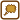## Diameter of Oleic Acid Molecule

### Task number: 2079

Approximate the diameter of an oleic acid C17H33COOH molecule using Avogadro’s constant. Assume that molecules are spherical and spaces between them make up 26 % z of the total volume(so called tight arrangement). The density of oleic acid is ρ = 900 kg·m−3.

• #### List of known information

 ρ = 900 kg m−3 oleic acid density 26 % of total volume spaces

From tables:

 NA = 6.023·1023 mol−1 Avogadr’s constant Ar(C) = 12.011 relative carbon atom mass Ar(H) = 1.0079 relative hydrogen atom mass Ar(O) = 15.999 relative oxygen atom mass
• #### Analysis

Avogadro’s constant tells us how many particles there are in one mole of matter. If we find out the volume of one mole of oleic acid, we can determine the volume of one molecule. Since we assume that their shape is spherical, we can calculate the volume easily from sphere volume equation.

We must know the density to determine the molar volume of the oleic acid. The density is given and the molar mass can be found using the relative atom masses of those atoms that make up the oleic acid. All can be found in tables.

We also have to consider that 26 % of the total volume are spaces between molecules, the molecules themselves have only 76 % of the molar volume.

• #### Hint 1 - molar mass

Using the relative atom masses of carbon, hydrogen and oxygen that can all be found in tables, determine the molar mass Mm of the oleic acid.

• #### Hint 2 - molar volume

Formulate the molar volume of the oleic acid using its molar mass and density and from there formulate the volume of one molecule using Avogadro’s constant. Don’t forget to subtract the volume of the spaces between molecules.

• #### Hint solution

Molar volume Vm is given by the formula

$V_\mathrm{m} = \frac{M_\mathrm{m}}{ρ},\tag{1}$

where Mm is the molar mass of the given matter and ρ is its density.

Spaces between molecules make up 26 % of the molar volume, the molecules themselves make up only 76 % of the molar volume. If we divide this volume by Avogadro’s constant (we divide the volume of one mole by the amount of particles in one mole), we will receive the volume of one molecule VO

$V_\mathrm{O} = \frac{0{,}74 V_\mathrm{m}}{N_\mathrm{A}}.\tag{2}$

The molecule has a spherical shape. Its volume is thus given by formula

$V_{O} = \frac{4}{3}πr^{3} = \frac{πd^{3}}{6},$

we express the diameter from the molecule’s volume

$d = \sqrt{\frac{6V_O}{π}}$

and we will use the equations (2) and (1) with this equation

$d = \sqrt{\frac{6·0.74V_\mathrm{m}}{πN_A}} = \sqrt{\frac{6·0.74M_\mathrm{m}}{πρN_\mathrm{A}}}.\tag{3}$
• #### Numerical substitution

We will substitute the molar mass, that we have calculated, into equation (3), the density from the assignment and Avogadro’s constant:

$d = \sqrt{\frac{6·0.74·M_\mathrm{m}}{πρN_\mathrm{A}}} \dot{=}\, \sqrt{\frac{6·0.74·2.86·10^{−1}}{900π·6.022·10^{23}}} \mathrm{m}$ $d \dot{=}\, 9.07 ·10^{−10} \mathrm{m}.$
• #### Answer

The diameter of an oleic acid molecule is approximately 9.07·10−10 m.

• #### Link to experiment

An experiment to measure the molecule’s diameter can be found here: Diameter of oleic acid molecule.×Original source: Inspirováno seminářem prof. E. Svobody. Zpracováno s podporou SFG (2016).